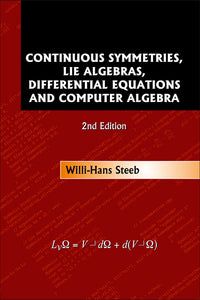# Continuous Symmetries, Lie Algebras, Differential Equations And Computer Algebra (2nd Edition)

Willi-hans Steeb

Format: Print Book

ISBN: 9789812708090

• SGD 180.83
Unit price per
Tax included.

This textbook comprehensively introduces students and researchers to the application of continuous symmetries and their Lie algebras to ordinary and partial differential equations. Covering all the modern techniques in detail, it relates applications to cutting-edge research fields such as Yang-Mills theory and string theory.Aimed at readers in applied mathematics and physics rather than pure mathematics, the material is ideally suited to students and researchers whose main interest lies in finding solutions to differential equations and invariants of maps.A large number of worked examples and challenging exercises help readers to work independently of teachers, and by including SymbolicC++ implementations of the techniques in each chapter, the book takes full advantage of the advancements in algebraic computation.Twelve new sections have been added in this edition, including: Haar measure, Sato's theory and sigma functions, universal algebra, anti-self dual Yang-Mills equation, and discrete Painlevé equations.

Format: Hardcover
No of Pages: 472
Imprint: World Scientific
Publication date: 20070803